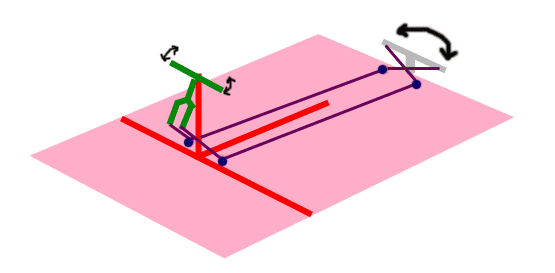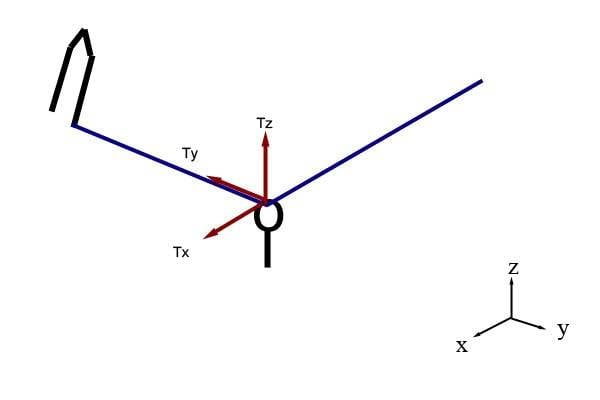# Force on a rope and a hook

#### gnittel

Hi guys! :)

1. The problem statement, all variables and given/known dataOk so this image may look funny, but this is actually an attempt to design a bike that can propel on water. What you are seeing is bike's handlebar (green), mounted on a support (red). Its supposed to have a rudder system at the back (gray). The pink rectangle is the wood where everything is placed (the raft). You can also see violet lines, this represent the rope to be pulled by the handlebars so the rudder would move along.

Now, the problem is to determine the optimum placement of the 4 hooks on the wood (represented by the blue dots) that will enable the driver to have an easier steering. Hooks are 1 inch in height.

the end of the fork (where one end of the rope is tied) is 12 inches above the surface of the wood.

Restrictions:

the front of the supports must be cleared from anything, so the hooks are to be placed only somewhere near the blue dot.

3. The attempt at a solution

Looking at one hook near the handle bars, I assigned a certain tension that is caused by the rudder (horizontal force) and a downward force by the hook. Problem is I dont think my process is correct. :(

Can someone tell me an good idea where to start? Thanks :)

#### nvn

Homework Helper
gnittel: You might want to define a vehicle coordinate system, such as +x forward, +y towards port side, and +z upward. There are two reaction components on the front hook (R1x and R1z). If T is cable tension, summation of horizontal and vertical forces on the front hook would be two equations and two unknowns (R1x and R1z). Therefore, you can solve for R1x and R1z.

#### gnittel

Hi nvn. Thanks for the reply.

My problem is I'm not really sure what forces a hook can contribute.

There are two reaction components on the front hook (R1x and R1z).
This is correct if we assume that looking at the front view of the vehicle, there is no difference in the y-axis locations of one end of the fork and one hook. But if I placed the hook at a different location, there should be a R1y right?

For example I placed the hook at a location where there will be all 3 components of tension,How would the reactions on the hook look like?

#### nvn

Homework Helper
gnittel: Yes, placing the hook at a location such that the cables are nonplanar is fine. You will then have three equations and three unknowns. By the way, let's change the name of the front hook reaction forces to RBx, RBy, RBz. (To save time, notice I am currently writing subscripts, such as Bx in RBx, as nonsubscripted.)

Let's say the cable is attached to the fork at point A; the front hook is at point B; and the rear hook is at point C. Here are the x, y, and z coordinates of points A, B, and C, right?

A(Ax, Ay, Az)
B(Bx, By, Bz)
C(Cx, Cy, Cz)

Therefore, see if the following makes sense. Below, notice, e.g., (Ax - Bx)/LBA = direction cosine of cable BA w.r.t. x axis.

LBA = cable BA length.
LBC = cable BC length.
TBA = tensile force in cable BA.
TBC = tensile force in cable BC.
RB = front hook reaction force (positive means positive x, y, or z axis direction).
RC = rear hook reaction force.
T = |TBA| = |TBC| = magnitude of cable tensile force.

LBA = [(Ax - Bx)^2 + (Ay - By)^2 + (Az - Bz)^2]^0.5.
LBC = [(Cx - Bx)^2 + (Cy - By)^2 + (Cz - Bz)^2]^0.5.
TBA = T*[(Ax - Bx)/LBA]*i + T*[(Ay - By)/LBA]*j + T*[(Az - Bz)/LBA]*k.
TBC = T*[(Cx - Bx)/LBC]*i + T*[(Cy - By)/LBC]*j + T*[(Cz - Bz)/LBC]*k.
RB = RBx*i + RBy*j + RBz*k.
RC = RCx*i + RCy*j + RCz*k.

Summation of forces at point B follows. Keep in mind, this is actually three equilibrium equations, because the i, j, and k components can be considered separately.

summation(FB) = 0 = TBA + TBC + RB.

Therefore, solve the above equilibrium equation for RB; i.e., solve for RBx, RBy, and RBz. Give it a try. Let me know if you think I made a typographic mistake anywhere. Note: I would probably recommend, draw RBx, RBy, and RBz in the positive x, y, and z directions, so that positive will mean positive global coordinate system (CS) direction, and negative will mean negative global CS direction.

#### nvn

Homework Helper
Do you plan to neglect friction in this problem? I have not really thought about friction yet. Friction could make this problem substantially more complicated. In my previous post, I assumed you are neglecting friction. Let me know if I misinterpreted.

#### gnittel

Yes nvn, I'm neglecting friction.

But I'm quite confused with the unknowns.

I separated the i, j and k components and used the equilibrium equation you posted.

i: T[(Ax-Bx)/LBA] + T[(Cx-Bx)/LBC] + RBx = 0
j: T[(Ay-By)/LBA] + T[(Cy-By)/LBC] + RBy = 0
k: T[(Az-Bz)/LBA] + T[(Cz-Bz)/LBC] + RBz = 0

So my unknowns are RBx, RBy and RBz. However, I dont get how I would solve for T.

#### nvn

Homework Helper
gnittel: The equations in post 6 are for obtaining the front hook reaction forces given T; i.e., after you obtain T.

To obtain T, you must analyze the rudder (and rudder arm) geometry, given the applied load on the rudder. The applied load on the rudder is due to water drag force, which is a function of rudder geometry, rudder angle, and vehicle velocity. Are you given the applied rudder force and moment, or are you given anything so you can compute the applied rudder force and moment?

#### gnittel

Oh I see. I think I need to assume some of the parameters you mentioned.

Having assumed the value of T, what would be my indicator that I have in fact found the optimum position of my hook?

Last edited:

#### nvn

Homework Helper
gnittel: Given any rudder applied force and/or moment, an indicator of optimum rear hook position would be minimum cable tensile force T. Given any value of T, an indicator of optimum front hook position would be minimum force applied by the driver. Is this a school assignment?

### The Physics Forums Way

We Value Quality
• Topics based on mainstream science
• Proper English grammar and spelling
We Value Civility
• Positive and compassionate attitudes
• Patience while debating
We Value Productivity
• Disciplined to remain on-topic
• Recognition of own weaknesses
• Solo and co-op problem solving